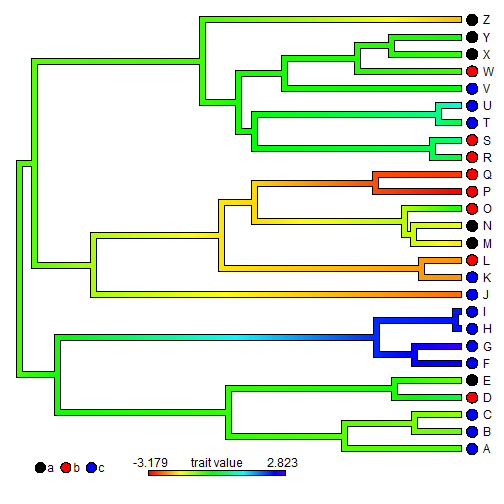## Tuesday, January 10, 2017

### Overlaying a `contMap` style continuous character map on a `dotTree` plot

A phytools user recently posted the following question:

“Is there a blog post / function capable of combining the functionality of contmap and dottree? I'm looking to plot a continuous character with a color ramp on the tree with a discrete character dot next to the tips of the tree. If I there is a post on this I have missed it in my search and would appreciate being pointed in the right direction if it is out there.”

The answer is that this is pretty straightforward to do - though it does take a little investigating of the internals of each function.

Here my approach will be to compute a `"contMap"` object & then overlay it on top of a plotted `dotTree`.

We can start by imagining the following data:

``````library(phytools)
tree
``````
``````##
## Phylogenetic tree with 26 tips and 25 internal nodes.
##
## Tip labels:
##  A, B, C, D, E, F, ...
##
## Rooted; includes branch lengths.
``````
``````x
``````
``````## A B C D E F G H I J K L M N O P Q R S T U V W X Y Z
## c c c b a c c c c c c b a a b b b b b c c c b a a a
## Levels: a b c
``````
``````y
``````
``````##       A       B       C       D       E       F       G       H       I
## -0.7592 -0.9114 -1.1249  0.0006 -0.8630  2.5855  2.8233  2.4789  2.3854
##       J       K       L       M       N       O       P       Q       R
## -2.5600 -2.3400 -2.3566 -1.8551 -1.6360 -0.4659 -3.1787 -2.8704 -0.0257
##       S       T       U       V       W       X       Y       Z
##  0.3514  0.0436  0.9419 -0.2918 -0.6332 -0.5828 -0.3969 -2.1122
``````

Next, we compute our `"contMap"` object, but we don't plot it:

``````obj<-contMap(tree,y,plot=FALSE)
obj
``````
``````## Object of class "contMap" containing:
##
## (1) A phylogenetic tree with 26 tips and 25 internal nodes.
##
## (2) A mapped continuous trait on the range (-3.1787, 2.8233).
``````

Finally, we plot our discrete character using `dotTree` after which we overlay our `contMap` style plot. This is the tricky part because I had to look up & duplicate the internal plotting parameters of `dotTree` to ensure that the two trees where directly overlain:

``````cols<-setNames(c("black","red","blue"),c("a","b","c"))
dotTree(tree,x,colors=cols,lwd=7)
par(fg="transparent")
ylim=c(-1/25*Ntip(tree),Ntip(tree)),offset=1.7)
par(fg="black")
lims=obj\$lims,digits=3,prompt=FALSE,x=0.3*max(nodeHeights(tree)),
y=0.4*(1+par()\$usr),lwd=4,fsize=1,subtitle="")
``````That's it!

1.2.